# MP Board Class 10th Maths Solutions Chapter 11 Constructions Ex 11.1

In this article, we will share MP Board Class 10th Maths Book Solutions Chapter 11 Constructions Ex 11.1 Pdf, These solutions are solved subject experts from the latest edition books.

## MP Board Class 10th Maths Solutions Chapter 11 Constructions Ex 11.1

In each of the following, give the justification of the construction also.

Question 1.
Draw a line segment of length 7.6 cm and divide it in the ratio 5 : 8. Measure the two parts.
Solution:
Steps of Construction :
I. Draw a line segment AB = 7.6 cm.
II. Draw a ray AX making an acute angle with AB.
III. Mark 13 = (8 + 5) equal points on AX, such that AX1 = X1X2 = ……….. X12X13.
IV. Join points X13 and B.
V. From point X5, draw X5C || X13B, which meets AB at C.
Thus, C divides AB in the ratio 5 : 8 On measuring the two parts, we get AC = 2.9 cm and CB = 4.7 cm.Justification:
In ∆ABX13 and ∆ACX5, we have
CX5 || BX13
∴ $$\frac{A C}{C B}=\frac{A X_{5}}{X_{5} X_{13}}=\frac{5}{8}$$ [By Thales theorem]
⇒ AC : CB = 5 : 8.

Question 2.
Construct a triangle of sides 4 cm, 5 cm and 6 cm and then a triangle similar to it whose sides are $$\frac{2}{3}$$ of the corresponding sides of the first triangle.
Solution:
Steps of Construction :
I. Draw a ∆ABC such that BC = 6 cm, AC = 5 cm and AB = 4 cm.
II. Draw a ray BX making an acute angle ∠CBX.
III. Mark three points [greater of 2 and 3 in $$\frac{2}{3}$$] X1, X2, X3 on BX1 such that BXj = X1X2 = X2X3.IV. Join X3C.
V. Draw a line through X2 such that it is parallel to X3C and meets BC at C’.
VI. Draw a line through C parallel to CA which intersect BA at A’.
Thus, ∆A’BC’ is the required similar triangle.
Justification :
By construction, we have X3C || X2C’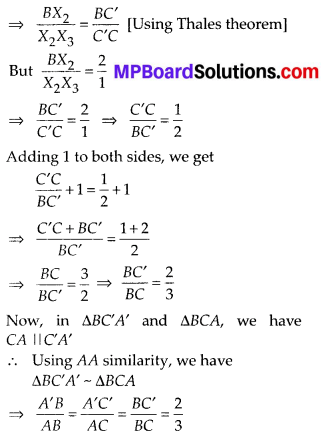Question 3.
Construct a triangle with sides 5 cm, 6 cm and 7 cm and then another triangle whose sides are $$\frac{7}{5}$$ of the corresponding sides of the first triangle.
Solution:
Steps of Construction :
I. Construct a ∆ABC such that AB = 5 cm, BC = 7 cm and AC = 6 cm.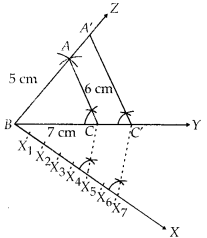II. Draw a ray BX such that ∠CBX is an acute angle.
III. Mark 7 points of X1, X2, X3, X4, X5, X6 and X7 on BX such that BX1 = X1X2 = X2X3 = X3X4 – X4X5 = X5X6 = X6X7
IV Join X5 to C.
V. Draw a line through X7 intersecting BC (produced) at C’ such that X5C || X7C’
VI. Draw a line through C’ parallel to CA to intersect BA (produced) at A’.
Thus, ∆A’BC’ is the required triangle.
Justification:
By construction, we have C’A’ || CA
∴ Using AA similarity, ∆ABC ~ ∆A’BC’Question 4.
Construct an isosceles triangle whose base is 8 cm and altitude 4 cm and then another triangle whose sides are $$1 \frac{1}{2}$$ times the corresponding sides of the isosceles triangle.
Solution:
Steps of Construction :
I. Draw BC = 8 cm
II. Draw the perpendicular bisector of BC which intersects BC at D.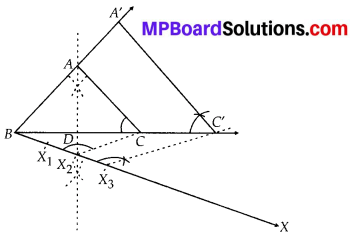III. Mark a point A on the above perpendicular such that DA = 4 cm.
IV. Join AB and AC.
Thus, ∆ABC is the required isosceles triangle.
V. Now, draw a ray BX such that ∠CBX is an acute angle.
VI. On BX, mark three points [greater of 2 and 3 in $$\frac{2}{3}$$] X1, X2 and X3 such that BX1 = X1X2 = X2X3
VII. Join X2C.
VIII. Draw a line through X3 parallel to X2C and intersecting BC (extended) to C’.
IX. Draw a line through C’ parallel to CA intersecting BA (extended) to A’, thus, ∆A’BC’ is the required triangle.
Justification:
We have C’A’ || CA [By construction]
∴ Using AA similarity, ∆A’BC’ ~ ∆ABCQuestion 5.
Draw a triangle ABC with side BC = 6 cm, AB = 5 cm and ∠ABC = 60°. Then construct a triangle whose sides are $$\frac{3}{4}$$ of the corresponding sides of the triangle ABC.
Solution:
Steps of construction :
I. Construct a ∆ABC such that BC = 6 cm, AB = 5 cm and ∠ABC = 60°.
II. Draw a ray BX such that ∠CBX is an acute angle.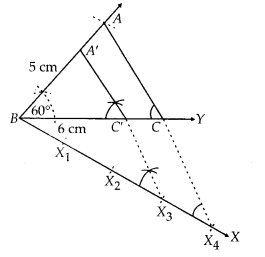Mark four points [greater of 3 and 4 in $$\frac{3}{4}$$] X1, X2, X3, X4 on BX such that 4
BX1 = X1X2 = X2X3 = X3X4
IV. Join X4C and draw a line through X3 parallel to X4C to intersect BC at C’.
V. Also draw another line through C’ and parallel to CA to intersect BA at A’.
Thus, ∆A’BC’ is the required triangle.
Justification:
In ∆BX4C we have
X4C || X3C’ [By construction]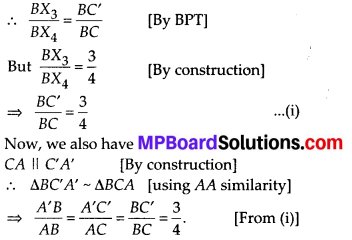Question 6.
Draw a triangle ABC with side BC = 7 cm, ∠B = 45°, ∠A = 105°.Then, construct a triangle whose sides are $$\frac{4}{3}$$ times the corresponding sides of ∆ABC.
Solution:
Steps of Construction :
I. Construct a AABC such that BC = 7 cm, ∠B = 45°, ∠A = 105° and ∠C = 30°
II. Draw a ray BX making an acute angle ∠CBX with BC.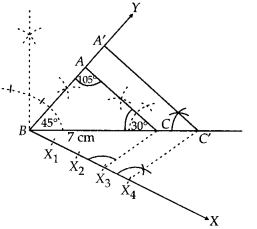III. On BX, mark four points [greater of 4 and 3 in $$\frac{4}{3}$$ ] X1, X2, X3 and X4 such that BX1 = X1X2 = X2X3 = X3X4.
IV. Join X3C.
V. Draw a line through X4 parallel to X3C intersecting BC(extended) at C’.
VI. Draw a line through C parallel to CA intersecting the extended line segment BA at A’.
Thus, ∆A’BC’ is the required triangle. Justification:
By construction, we have
C’A’ || CA
∴ ∆ABC ~ ∆A’BC’ [AA similarity]Question 7.
Draw a right triangle in which the sides (other than hypotenuse) are of lengths 4 cm and 3 cm. Then construct another triangle whose sides are $$\frac{5}{3}$$ times the corresponding sides of the given triangle.
Solution:
Steps of Construction :
I. Construct the right triangle ABC such that ∠B = 90°, BC = 4 cm and BA = 3 cm.
II. Draw a ray BX such that an acute angle ∠CBX is formed.
III. Mark 5 points X1, X2, X3, X4 and X5 on BX such that BX1 = X1X2 = X2X3 = X3X4 = X4X5.
IV. Join X3C.
V. Draw a line through X5 parallel to X3C, intersecting the extended line segment BC at C’.
VI. Draw another line through C’ parallel to CA intersecting the extended line segment BA at A’.
Thus, ∆A’BC’ is the required triangle.
Justification:
By construction, we have C’A’ || CA
∴ ∆ABC ~ ∆A’BC’ [AA similarity]